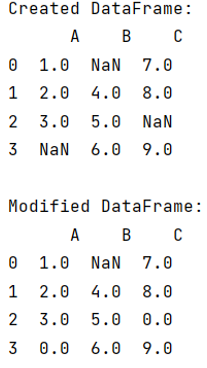# Pandas dataframe fillna() only some columns in place

Given a Pandas DataFrame, we have to use fillna() only some columns in place.
Submitted by Pranit Sharma, on June 07, 2022

## pandas.DataFrame.fillna() Method

This method is used to replace the null values with a specified value which is passed as a parameter inside this method.

Syntax:

```DataFrame.fillna(
value=None,
method=None,
axis=None,
inplace=False,
limit=None,
downcast=None
)
```

To apply this method to specific columns, we need to define the specific columns at time of function calling.

To work with pandas, we need to import pandas package first, below is the syntax:

```import pandas as pd
```

Let us understand with the help of an example,

```# Importing pandas package
import pandas as pd

# Importing numpy package
import numpy as np

# Creating a dictionary
d = {
'A':[1,2,3,np.NaN],
'B': [np.NaN,4,5,6],
'C':[7,8,np.NaN,9]
}

# Creating an empty DataFrame
df = pd.DataFrame(d)

# Display DataFrame
print("Created DataFrame:\n",df,"\n")

# Filling 0 in place of NaN values
df[['A','C']] = df[['A','C']].fillna(value=0)

# Display modified DataFrame
print("Modified DataFrame:\n",df)
```

Output:What's New (MCQs)

Top Interview Coding Problems/Challenges!

IncludeHelp's Blogs

Languages: » C » C++ » C++ STL » Java » Data Structure » C#.Net » Android » Kotlin » SQL
Web Technologies: » PHP » Python » JavaScript » CSS » Ajax » Node.js » Web programming/HTML
Solved programs: » C » C++ » DS » Java » C#
Aptitude que. & ans.: » C » C++ » Java » DBMS
Interview que. & ans.: » C » Embedded C » Java » SEO » HR
CS Subjects: » CS Basics » O.S. » Networks » DBMS » Embedded Systems » Cloud Computing
» Machine learning » CS Organizations » Linux » DOS
More: » Articles » Puzzles » News/Updates